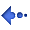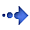Water Rocket Computer Model Equations

Computer models

Why not use a straight forward mathematical equation and save all of this hassle?

Some equations provide straight answers to simple questions such as how far will something have travelled after a particular amount of time has elapsed? The equation s = ut + ½at² gives the answer to this where: s = the distance; u = the initial velocity; t = the time interval; and, a = the acceleration. If you pick the right units, there will be no awkward constants - SI units are good enough for this. This is fine for rockets that only lose a little of their mass during the burn stage such as the solid fuel rockets used in toys and for these, there is Randy Culp's excellent page at http://www.execpc.com/~culp/rockets/rckt_eqn.html which gives details of solid fuel rocket flight equations - the larger solid fuel rockets, such as the two on the space shutte, are able to carry a greater proportion of fuel therefore suffering the same problems with calculations as the water rockets. With water rockets (as the big solid fuel boosters), around 90% of the weight disappears through the nozzle during the 'burn' (86% with the space shuttle boosters I am reliably told). The problem arises when you realise that in a water rocket, the acceleration is far from constant, changing all of the way through the 'burn' part of the flight and disappearing altogether after that. Figures for average mass or acceleration do not work in this case.

Clearly, for water rockets, a model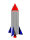that works out what is going to happen in the next few milliseconds and recalculate then, repeating until the flight is over, is going to give a clearer picture of what is going to happen. Each time interval produces a path to the next set of calculations and therefore, the closer together these are, the less error there is going to be. Besides, You would need a PhD in mathematics and at least several decades of post doctoral experience (including at least one ground-breaking discovery into possibly a new branch of mathematics) to produce a meaningful single equation if it is possible at all.

So, it looks like we are stuck with a computer model.

Considerations

Momentum and Drag

A rocket is essentially a tank that is propelled upwards by the ejection of some mass. The acceleration of the ejected material produces an equal reaction in the opposite direction - pushing matter downwards, out of a rocket exhaust produces a reaction that tries to push the rocket upwards. During the course of the flight, the rockets momentum keeps it going until drag and gravity overcome it. In the case of a water rocket, the reaction mass is water and the force that is used to push is out comes from the pressure of the compressed air inside the rocket.

The equation F = ma where F = the force; m = the mass; and, a = the acceleration may be used to calculate the acceleration of the rocket remembering that the forces balance each other out so that the mass of the reaction mass x  the acceleration of the reaction mass = the mass  of the rocket x the acceleration of the rocket or m pap = mrar

However, this is not the complete picture as there are other forces on the rocket and it is simpler to group them together into upward and downward forces and perform a final sum.

The other force considered that acts on the rocketis drag - usually considered to be proportional to the square of the velocity. The actual amount of drag depends upon the shape of the rocket and therefore cannot be calculated easily - as it is a combination of a number of quite complicated factors that all remain constant, it is usually lumped together into a single number. It is better to have a nominal drag factor that is then tested against the real model and modified until the computer model agrees with the real rocket - in this way the computer model becomes more accurate (it is easier to change a flimsy and complicated constant in a computer model than alter the laws that operate the Universe).

Flow of Reaction Mass

One other thing to consider is how do we calculate the speed of the water (so that we can calculate its contribution to the momentum of the rocket). We know that the pressure inside the rocket forces out the water so we need to have an understanding of the way that gasses perform as well as how pressure is used to force a fluid through a pipe. As the water is forced out, the gas expands and the pressure drops - we need to know how much this happens to be able to predict the pressure for a given volume.

Gas

 Everyone knows that if you compress a gas, the pressure will increase but by how much? The Ideal Gas Law states . . .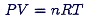where . . .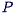is the Pressure;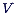is the Volume;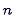is the number of moles of gas;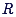is the Universal Gas Constant; and,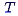is the Absolute Temperature (K). which is all right if you know what you are starting with - we do but we do not know how P and V are related. Under isothermal conditions . . .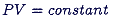but the burn part of the flight is so short that there is no change for heat to travel into the rocket to warm up the gas so adiabatic expansion has to be assumed (there is no reason to suspect that reality is that far from adiabatic expansion) thus . . .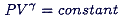where . . .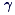is the ratio of specific heats such that . . .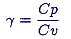where . . .is the specific heat capacity at constant pressure; and,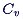is the specific heat capacity at constant volume.is usually fixed at 1.4 Once all of the water has escaped from the water rocket, the gas pressure is used to calculate the air impulse - the air escaping from the nozzle will provide some extra thrust.

Fluid

I say fluid because it doesn't really matter if it is water or something else - try sea water.

In a vertical system, the interface between the air and the water may be considered as a piston and any gas dissolving in the water ignored (Carbon Dioxide will dissolve and reduce the pressure in the rocket until it reaches equilibrium and if there is enough, it will effervesce as it depressurises upon passing through the nozzle making the viscosity and flow characteristics unpredictable).

There are calculations that relate to the viscosity of a fluid but it has been found out by experiment that these effects, caused by turbulent flow, do not have a chance to establish themselves in the short distances operating in the nozzle of a water rocket - the pressure losses due to friction loss being negligable. The concentration of flow does, however, reduce the effective pressure drop along the nozzle and this does creep into the calculation as a constant dependent upon the internal geometry of the nozzle - a little like a drag factor but for the inside of the rocket.

Knowing the velocity, we can calculate the mass of reaction mass and its contribution to the momentum of the rocket system. By calculating through a set of equations, we are able to work out where in the sky the rocket will be, how fast it is travelling and various other values.

Limitations

For a water rocket designed in the middle of the effective range, with all calibrations perfected, the flight should be predicted correctly. However, errors start to creep into things throughout the process when there is too great a deviation from this calibrated norm.

For the distances and speeds travelled, Newtonian mechanics is good enough - we are hardly likely to encounter a significant manifestation of the twins paradox with a water rocket (it actually works out to be around 4fs that is 0.000 000 000 000 004 seconds accumulated time dilation over the trip; for anyone looking upwards at its maximum speed, gives a red shift of around 0.1ppm; and, applying the Lorentz transformation makes its length contract by around 1fm for the stationary observer although the effects of external air pressure on the rocket body during flight will make it shrink more than this).

For it to work, Water, travelling at velocity 1 is accelerated to velocity 2. If the ratio of the diameters of the body of the rocket to the nozzle is sufficiently high, then velocity 1 may be ignored. However, the model takes this into account therefore smaller constriction ratios may be used. This means that the thrust will vary according to the dry weight of the rocket because the lighter the rocket, the more the acceleration, the greater the pressure at the nozzle, the greater the thrust, the more the acceleration . . .

Just to make this more interesting, the air on the outside is also: compressible; changes its pressure and temperature (and viscosity) at different heights; and; has movement in it. Hmmph.The equations themselves make certain assumptions: assuming that the equations describe accurately the effects of pressure on the fluid; the fact that the rocket will change its size when the pressure inside it alters during flight along with the pressure on the front of the rocket that causes drag; the fact that the ratio of specific heat capacity at constant pressure and volume is not a constant at all the effect of the vapour pressure of the reaction mass on the dew point of the gas and its effect on the specific heat capacities; the fact that the coefficient of drag changes slightly over the range of speeds of a water rocket; and so on.

Many of these effects are small and can be ignored - the errors encountered when flying the real rocket will by far exceed any of these. Working out the pressure that you deliver to the rocket is a problem if you have not got a pressure gauge.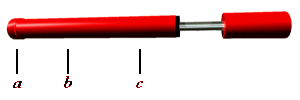You know that the end of the bicycle pump (in the diagram) is at point a and that you started the pump stroke at point c and that the one-way valve started letting air through at point b and from this, you can work out the pressure in the rocket if you take into account the fact that you don't have a particularly good idea of where the piston really is and you don't know what pressure is needed to open the one-way valve. Did you really need to know the height of the flight to the nearest foot when, during the real flight, it shot off at 45° and landed in a neighbour's garden? or that you forgot to make sure that the water inside was still and a vortex appeared allowing all of the gas to escape with 20%of the water still inside it? There is plenty to go wrong with launching water rockets - I can vouch for that - getting a perfect first flight turned out to be a fluke that wan't repeated until about flight six. A rule to remember is that if anything goes wrong, the flight will be low and short, and probably land in a neighbour's garden or up a tree.

For discussion on the topic of improving flights - assembly of more advanced rockets, glues, lauchers (a swing arm launcher can't possibly be as dangerous as it sounds) delivery of compressed gas, release mechanisms and so on, join in on e-mail discussion on http://www.h2orocket.com/email.html or http://www.osa.com.au/~cjh/rockets/list.html

In Summary

We can perform complex calculations that are unfeasible to express as a single equation by following a series of logical steps which go as follows . . .

1. 2. Work out the initial conditions and any constants that will speed up any of the subsequent calculations. Work out the air pressure. Work out the flow of reaction mass Work out the new mass of the system (remember that you have lost some out of the back of the rocket). Work out the drag of the system (based upon the previous velocity of the system) net forces and then the acceleration of the system Work out the velocity of the system. Check if any reaction mass left if yes, go to step 2 Check if any pressure left if yes, go to step 2 Check still off ground if yes, go to step 5 End.

Hopefully, this process allows the real water rocket's flight to be predicted reasonably accurately. Put in a parachute and it looks a little more complicated than this :-)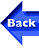Back to the Water Rocket Computer Model pageBack to the Index Copyright 1998 - 2003 P.A.Grosse. All Rights Reserved